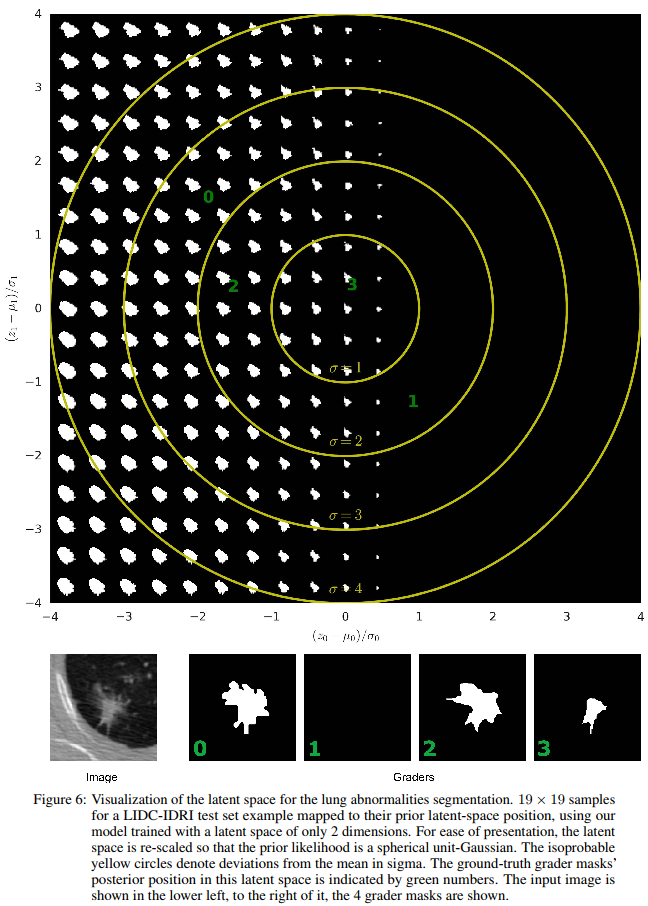## The idea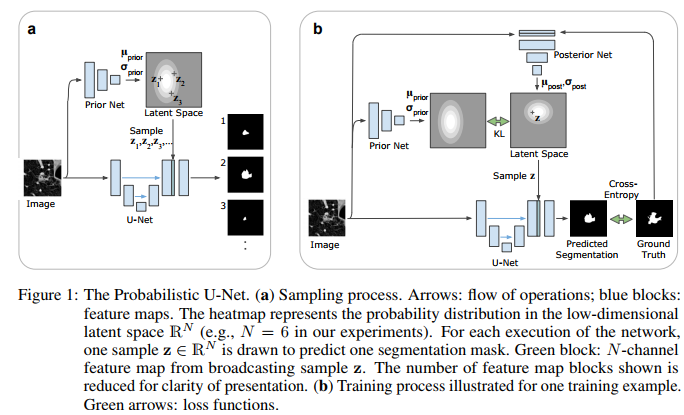In this paper, the authors present a stochastic U-Net-based segmentation method capable of grasping the inherent ambiguities of certain segmentation applications. In a nutshell, by its stochastic nature, for one given image, the system can produce a wide variety of segmentation maps that mimic what several humans would manually segment.

## The method

The proposed system is very well illustrated in figure 1. The fundamental hypothesis this paper is based upon is that the label maps produced by human annotators are governed by a latent variable z. Since neither $P(z|X)$ nor $P(Y|x)$ are known a priori (X: Input image, Y: Output segmentation), they rely on a Conditional Variational Autoencoder (VAE) to learn it. The proposed system at test time is illustrated in Fig.1(a) and a train time in Fig.1(b). The stochastic nature of the system is driven by random latent variables $z$ sampled in the latent space: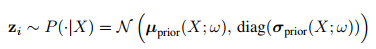which is then appended at the end of the U-Net: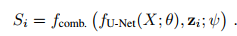The main difference with a conventional VAE is that the prior distribution of the latent variables $P(z)$ is not a simple Gaussian distribution. It is instead a conditional distribution $P(z|X,Y)$ learned by a posterior net (fig.1b) hence why the VAE is said to be conditional. At training time, $z$ is sampled with the posterior net: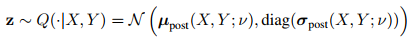and the overall loss is as follows: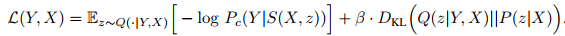.

## The results

They compare their method to the 4 stochastic segmentation methods shown in Fig.2.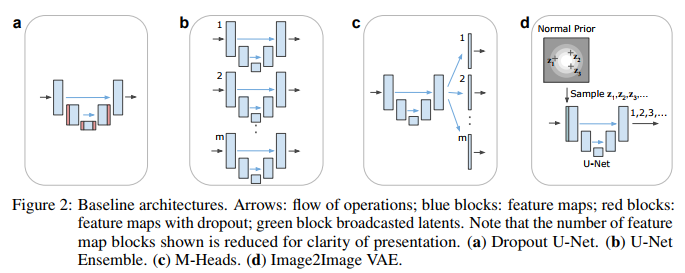They tested their method on two datasets : a CT-Scan lung dataset and the Cityscape dataset. They show that in both case, their method is better on average (Fig.4)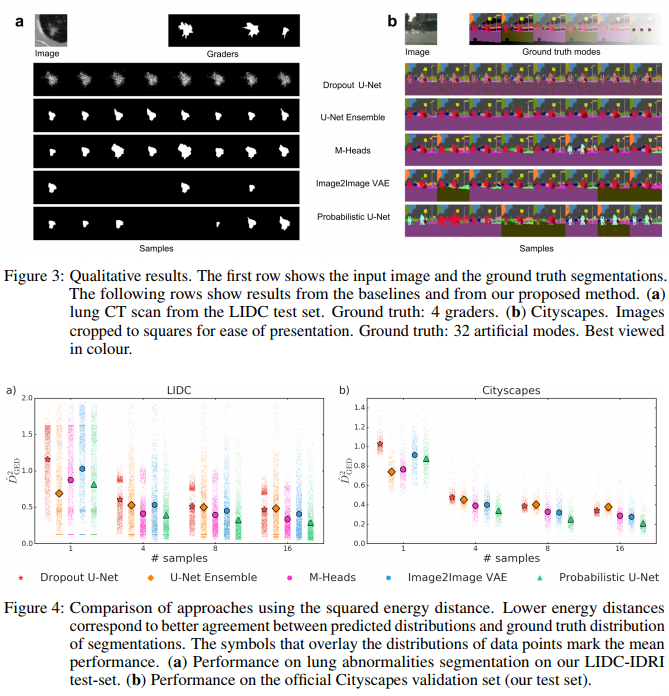They also show through an interesting figure that the learned latent space does grasp the variability of human annotators.